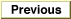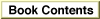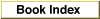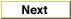Legacy DocumentImportant: The information in this document is obsolete and should not be used for new development.

Inside Macintosh: QuickDraw GX Environment and Utilities /
Chapter 8 - QuickDraw GX Mathematics / Using QuickDraw GX Mathematics

Shifting the Bits of a wide Number

You can use the WideShift function to shift bits in a wide format number. Listing 8-3 shows how to use the WideShift function to provide a fixed-point version of the VectorMultiply function.

Listing 8-3 Using the WideShift function to create a fixed-point VectorMultiply function

Fixed VectorFixMul(long count, Fixed *vector1, long step1,
Fixed *vector2, long step2)
{
wide temp;
return WideShift(VectorMultiply(count, vector1, step1,
vector2, step2, &temp), 16)->lo;
}
Listing 8-4 shows how to use the WideShift function in a multiplication function for a fixed-point number with a fixed-point bias of 6 bits.

Listing 8-4 Using the WideShift function in a fixed-point multiplication function

long MultiplyDot6(long a, long b)
{
wide temp;
return (long)WideShift(WideMultiply(a, b, &temp), 6)->lo;
}
Listing 8-5 shows how to use the WideShift function in a division function for a fixed-point number with a fixed-point bias of 6 bits. Listing 8-6 gives an alternative, but equivalent, approach.

Listing 8-5 Using the WideShift function to create a fixed-point division function

long DivideDot6(long a, long b)
{
wide temp;
temp.hi = (temp.lo = a) < 0 ? -1 : 0;  /* sign extend a */
return WideDivide(WideShift(&temp, -6), b, 0);
}
Listing 8-6 shows how to use the WideShift function for a second fixed-point division function with a fixed-point bias of 6 bits. Listing 8-5 gives an alternative, but equivalent, approach.

Listing 8-6 Using the WideShift function to create a second fixed-point division function

long DivideDot6(long a, long b)
{
wide temp;
temp.hi = a;
temp.lo = 0;
return WideDivide(WideShift(&temp, 26), b, 0);
}